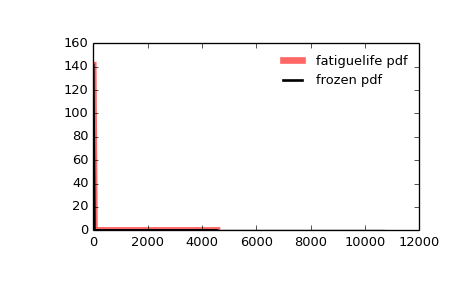# scipy.stats.fatiguelife¶

scipy.stats.fatiguelife = <scipy.stats._continuous_distns.fatiguelife_gen object at 0x4503ec8c>[source]

A fatigue-life (Birnbaum-Saunders) continuous random variable.

As an instance of the rv_continuous class, fatiguelife object inherits from it a collection of generic methods (see below for the full list), and completes them with details specific for this particular distribution.

Notes

The probability density function for fatiguelife is:

fatiguelife.pdf(x, c) =
(x+1) / (2*c*sqrt(2*pi*x**3)) * exp(-(x-1)**2/(2*x*c**2))


for x > 0.

fatiguelife takes c as a shape parameter.

The probability density above is defined in the “standardized” form. To shift and/or scale the distribution use the loc and scale parameters. Specifically, fatiguelife.pdf(x, c, loc, scale) is identically equivalent to fatiguelife.pdf(y, c) / scale with y = (x - loc) / scale.

References

 [R314] “Birnbaum-Saunders distribution”, http://en.wikipedia.org/wiki/Birnbaum-Saunders_distribution

Examples

>>> from scipy.stats import fatiguelife
>>> import matplotlib.pyplot as plt
>>> fig, ax = plt.subplots(1, 1)


Calculate a few first moments:

>>> c = 29
>>> mean, var, skew, kurt = fatiguelife.stats(c, moments='mvsk')


Display the probability density function (pdf):

>>> x = np.linspace(fatiguelife.ppf(0.01, c),
...                 fatiguelife.ppf(0.99, c), 100)
>>> ax.plot(x, fatiguelife.pdf(x, c),
...        'r-', lw=5, alpha=0.6, label='fatiguelife pdf')


Alternatively, the distribution object can be called (as a function) to fix the shape, location and scale parameters. This returns a “frozen” RV object holding the given parameters fixed.

Freeze the distribution and display the frozen pdf:

>>> rv = fatiguelife(c)
>>> ax.plot(x, rv.pdf(x), 'k-', lw=2, label='frozen pdf')


Check accuracy of cdf and ppf:

>>> vals = fatiguelife.ppf([0.001, 0.5, 0.999], c)
>>> np.allclose([0.001, 0.5, 0.999], fatiguelife.cdf(vals, c))
True


Generate random numbers:

>>> r = fatiguelife.rvs(c, size=1000)


And compare the histogram:

>>> ax.hist(r, normed=True, histtype='stepfilled', alpha=0.2)
>>> ax.legend(loc='best', frameon=False)
>>> plt.show()Methods

 rvs(c, loc=0, scale=1, size=1, random_state=None) Random variates. pdf(x, c, loc=0, scale=1) Probability density function. logpdf(x, c, loc=0, scale=1) Log of the probability density function. cdf(x, c, loc=0, scale=1) Cumulative density function. logcdf(x, c, loc=0, scale=1) Log of the cumulative density function. sf(x, c, loc=0, scale=1) Survival function (1 - cdf — sometimes more accurate). logsf(x, c, loc=0, scale=1) Log of the survival function. ppf(q, c, loc=0, scale=1) Percent point function (inverse of cdf — percentiles). isf(q, c, loc=0, scale=1) Inverse survival function (inverse of sf). moment(n, c, loc=0, scale=1) Non-central moment of order n stats(c, loc=0, scale=1, moments='mv') Mean(‘m’), variance(‘v’), skew(‘s’), and/or kurtosis(‘k’). entropy(c, loc=0, scale=1) (Differential) entropy of the RV. fit(data, c, loc=0, scale=1) Parameter estimates for generic data. expect(func, c, loc=0, scale=1, lb=None, ub=None, conditional=False, **kwds) Expected value of a function (of one argument) with respect to the distribution. median(c, loc=0, scale=1) Median of the distribution. mean(c, loc=0, scale=1) Mean of the distribution. var(c, loc=0, scale=1) Variance of the distribution. std(c, loc=0, scale=1) Standard deviation of the distribution. interval(alpha, c, loc=0, scale=1) Endpoints of the range that contains alpha percent of the distribution

scipy.stats.f

scipy.stats.fisk